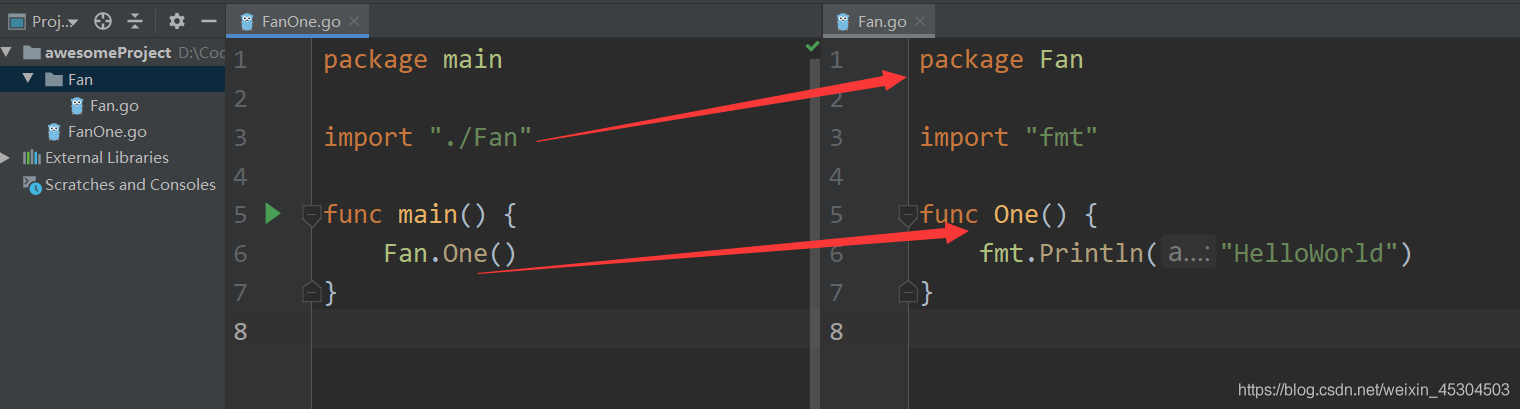﻿ Go语言七篇入门教程二程序结构与数据类型_Golang_脚本之家
Golang# Go语言七篇入门教程二程序结构与数据类型

## 1. 程序结构

### 1.1 名称```func main() {
FanOne := "666" //大驼峰 （注意！一般函数内部都是用小驼峰，局部变量，大驼峰一般用于函数的命名，需要外包的导出）
fanOne := "666" //小驼峰
}
```### 1.2 声明

go语言可通过`var`进行变量的声明

var 变量名 类型

```func main(){
var fanOne string
var xiaoSheng int
var a,b,c = true , 6.6 , "fanOne" //bool ,float , string
}
```

```func main(){
fanOne:="666" //string字符串
xiaoSheng := 666  //int整型
a,b,c := true , 6.6 ,"fanOne"  //bool ,float , string
}
```

### 1.3 注释

```//单行注释

/*

*/
```

### 1.4 单双引号

```func main(){
var d rune
d = '1'
a := 'a'
b := "a"
c := `'a'':"b"`  //可以把单引号和双引号整括起来
fmt.Printf("%T\n",d)	 // int32
fmt.Printf("%T\n",a)	 // int32
fmt.Printf("%T\n",b)	 // string
fmt.Printf("%T\n",c)  	 // string
}
```

### 1.5 输出

```//Println 自带换行 Printf 格式化输出

```## 2. 数据类型

### 2.1 整型

• 不同位数的整型区别在于能保存整型数字范围的大小；
• 有符号类型可以存储任何整数，无符号类型只能存储自然数
• int和uint的大小和系统有关，32位系统表示int32和uint32，如果是64位系统则表示int64和uint64
• byte与uint8类似，一般用来存储单个字符

### 2.2 浮点型

`float64``float32` 两种

• float64的精度要比float32的要准确
• 如果我们要保存一个精度高的数，则应该选择float64

Go的浮点型默认为`float64`类型

### 2.3 复数

`complex64``complex128` ， 二者分别由`float32``float64` 构成，内置的complex函数根据给定的实部和虚部创建复数，而内置的real函数和img函数则分别提取复数的实部和虚部：

```var x complex128 = complex(1,2) //1+2i
var y complex128 = complex(3,4) //3+4i
fmt.Println(x*y) 		//-5+10i
fmt.Println(real(x*y))  //-5
fmt.Println(imag(x*y))  //10
```

```x := 1 + 2i
y := 3 + 4i
```

### 2.4 布尔型

```var fanOne true
var xiaoSheng false
```

### 2.5 字符串

```func main(){
s:="FanOne"
fmt.Println(len(s)) //6
fmt.Println(s[:3])  //Fan
fmt.Println(s,s) // 97 110
}
```

```func main(){
s:="FanOne"
b := "666"
y := s + b
fmt.Println(y) //FanOne666
}
```

```num,err:=strconv.Atoi("666")//num就是整型
```

int 转 字符串

```str := strconv.Itoa(666) //str是字符串
```

### 2.6 常量

```const a = 666
fmt.Println(a) //666
```

### 2.7 数组

```var a int //3个整数的数组
for i , v := range a {
fmt.Println(i,v)
}
```
```var fan int{1,2,3}
var one int{1,3}
t := [...]int{1,2,3} //省略号
fmt.Printf("%T",t)	 //int
```

go语言的数组的长度是固定的，所以在某些场景下数组存在着它的局限性

### 2.8 切片

```// make只传入一个参数指定长度，则容量和长度相等。以下输出："len: 10, cap: 10"
s := make([]int, 10)
fmt.Printf("len: %d, cap: %d\n", len(s), cap(s))

// make 传入长度和容量。以下输出："len: 10, cap: 15"
s := make([]int, 10, 15)
fmt.Printf("len: %d, cap: %d\n", len(s), cap(s))

// 不允许创建容量小于长度的切片。下面语句编译会报错："len larger than cap in make([]int)"
s := make([]int, 10, 5)
```

```// 通过字面量声明切片，其长度和容量都为5。以下输出：“len: 5, cap: 5”
s := []int{1, 2, 3, 4, 5}
fmt.Printf("len: %d, cap: %d\n", len(s), cap(s))

// 可以在声明切片时利用索引来给出所需的长度和容量。
// 通过指定索引为99的元素，来创建一个长度和容量为100的切片
s := []int{99: 0}
```

```len = hith - low
cap = max - low
s1 := baseStr[1:3:10]
fmt.Printf("len: %d, cap: %d\n", len(s1), cap(s1)) // len: 2, cap: 9

s2 := baseStr[1:3]
fmt.Printf("len: %d, cap: %d\n", len(s2), cap(s2)) // len: 2, cap: 9

s3 := baseStr[:3]
fmt.Printf("len: %d, cap: %d\n", len(s3), cap(s3)) // len: 3, cap: 10

ss1 := s1[2:5]
ss2 := s1[3:8]
fmt.Printf("len: %d, cap: %d\n", len(ss1), cap(ss1)) // len: 3, cap: 7
fmt.Printf("len: %d, cap: %d\n", len(ss2), cap(ss2)) // len: 5, cap: 6
```

```// 创建一个整型切片
// 其长度和容量都是5个元素
slice := []int{1, 2, 3, 4, 5}

// 创建一个新切片
// 其长度为2 个元素，容量为4个元素
newSlice := slice[1:3]

// 使用原有的容量来分配一个新元素
// 将新元素赋值为 60
newSlice = append(newSlice, 6)

fmt.Printf("slice: %v\n", slice)       // slice: [1 2 3 6 5]
fmt.Printf("newSlice: %v\n", newSlice) // newSlice: [2 3 6]
```

### 2.9 map

`Map` 是一种无序的键值对的集合。Map 最重要的一点是通过 key 来快速检索数据，key 类似于索引，指向数据的值。

Map 是一种集合，所以我们可以像迭代数组和切片那样迭代它。不过，Map 是无序的，我们无法决定它的返回顺序，这是因为 Map 是使用 hash 表来实现的。

```/* 声明变量，默认 map 是 nil */
var map_variable map[key_data_type]value_data_type
/* 使用 make 函数 */
map_variable := make(map[key_data_type]value_data_type)
```
```	var fan map[string]string //创建集合
fan = make(map[string]string)
//map插入key-value对
fan [ "One" ] = "666"
fan [ "Four" ] = "999"
//使用键输出
for value := range fan {
fmt.Println(value, "is", fan [value])
}
//查看元素在集合中是否存在
val, ok := fan [ "Two" ] //如果确定是真实的,则存在,否则不存在
if ok {
fmt.Println("fanTwo is", val)
} else {
fmt.Println("fanTwo not exist")
}
```

delete() 函数用于删除集合的元素, 参数为 map 和其对应的 key。

```delete(fan , "One")
```

### 2.10 结构体

```type Person struct{
name string
age int
sex string
}
func main(){
person := Person{  //初始化
name: "fanOne",
age:  16,
sex:  "male",
}
fmt.Println(person.name)  //引用
}

```

### 2.11 JSON

```type Person struct{
Name string `json:"name"` //序列化成string类型
Age int	`json:"age"`
Sex string `json:"sex"`
}
func main(){
person := &Person{} //创建一个对象
var data = `{"name":"fanOne","age":"11","sex":"male"}`
_ = json.Unmarshal([]byte(data), &person)  //将这个data序列化成person的结构体，并传入其中
fmt.Println(person.Name)
}
```

## 3. 流程控制

### 3.1 条件语句

```func main(){
x :=1
if x == 1 {
fmt.Println("666")
} else {
fmt.Println("999")
}
}
```

### 3.2 选择语句

```	switch i {
case 0:
fmt.Printf("0")
case 1:
fmt.Printf("1")
case 2:
fmt.Printf("2")
case 3:
fmt.Printf("3")
case 4, 5, 6:
fmt.Printf("4, 5, 6")
default:
fmt.Printf("Default")
}
```

### 3.3 循环语句

```	sum := 0
for i := 0; i < 10; i++ {
sum += i
}

```
```	s := int{1,2,3}
for _,item := range s{
fmt.Println(item)
}
```